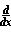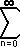# Math2.org Math Tables:(Math)
 Trig Function Topicsoverviewthe functionsunit modes - degree, radian, gradlabeling sides - opposite, adjacent, hypotenuse Trig Functions: Sine and Cosine Definition Definition: An algebraic approach From defining a few general properties of the sine and cosine functions, we can alegbraically derive the sine and cosine functions themselves. First, define the sine and cosine functions to have these properties:sin x = cos xcos x = - sin x sin 0 = 0 cos 0 = 1 Now, write the sine function as an arbitrary power series, in that let sin x = a0x0 + a1x1 + a2x2 + a3x3 + a4x4 + ...     sin 0 = 0 = a0           a0 = 0 By differentiating the power series and equating it with the cosine by the original properties,sin x = cos x = 0 + (1)a1x0 + (2)a2x1 + (3)a3x2 + (4)a4x3 + ...     cos 0 = 1 = (1)a1             a1 = 1 Continuing,cos x = -sin x = -(0 + 0 + (2)(1)a2x0 + (3)(2)a3x1 + (4)(3)a4x2 + ...)     -sin 0 = 0 = -(2)a2              a2 = 0-sin x = -cos x = -(0 + 0 + 0 + (3)(2)(1)a3x0 + (4)(3)(2)a4x1 + ...)      -cos 0 = -1 = (3)(2)(1)a3               a3 = -1/3!-cos x = sin x = 0 + 0 + 0 + 0 + (4)(3)(2)(1)a4x0 + ...     sin 0 = 0 = (4)(3)(2)(1)a4             a4 = 0 Continue on and you get values of all an    if n is even then an = 0    if n is odd then an = 1/n! alternating positive and negitive. or stated as:    a2n = 0    a2n+1 = (-1)n/(2n+1)! Pluging these values into the equation for sine:     sin x = x1/1! - x3/3! + x5/5! - x7/7! + x9/9! - ... + (-1)nx2n+1/(2n+1)! + ...           =(-1)nx2n+1 / (2n+1)! and for cosine:     cos x = 1 - x2/2! + x4/4! - x6/6! + x8/8! - ... + (-1)nx2n/(2n)! + ...           =(-1)nx2n / (2n)! These series converge for all x ÎÂ. Source: Jeff Yates.